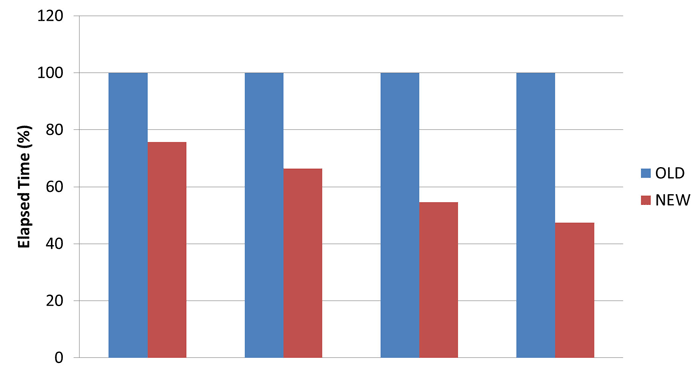# Improved Runtime

Developers at Flow Science are constantly working to improve the performance of our solver. We want to provide our users the same experience, i.e., accuracy and ease of use of FLOW-3D and FLOW-3D CAST, but with shorter runtimes. In the upcoming releases – FLOW-3D v11.1 and FLOW-3D CAST v4.1 – the results are the same, but the computational time is significantly reduced.

## Performance and Optimization

Before I jump into the results, I would like to provide some insights into the typical process of performance optimization of a code. Performance analysis involves finding the bottlenecks – the parts of the code that slow down the calculations the most. Code profiling techniques help us identify if a specific function has performance issues.

For example, we have three functions in our code – funcA, funcB and funcC. When we profile the code we know how much runtime is spent in each of these functions. Let’s say that funcA eats up 90% of the runtime, while the other two functions take the remaining 10%. In such a case, it only makes sense to target funcA. We can target other functions, but the returns on optimizing funcA will give the best results.

## Finding the Bottleneck

Profiling showed that for most applications the pressure solver in FLOW-3D was the most computationally intensive component (typically about 30-40% of total simulation time). Also, for Fluid Structure Interaction (FSI) problems, the FSI solver was found to consume a large chunk (70-80%) of the total computational time, dwarfing even the time spent in the pressure solver. Because the pressure solver is used more widely, we decided it would benefit more of our users to optimize it first.

## Optimizing the Solver

Optimization is the second step towards improving the overall runtime of the code. While there are many techniques involved in optimizing a certain segment of the code, the most important ones for this development were:

• Optimizing vector-vector calculations
• Optimizing matrix-vector calculations
• Storing the sparse matrix in an efficient and compact way

## Improvements in Runtime

Different tests were conducted after the optimizations were made. They are represented by the bar graphs below.Figure 1. Computational time for a 1.3 million cell weir simulation with FLOW-3D v11.1

In Figure 1, ITMIN is the minimum number of iterations of the pressure solver. Irrespective of the number of iterations there is a decrease in elapsed time compared to the original solver. But as the number of iterations increase, the performance gains are more significant. For ITMIN=10, the elapsed time has dropped down by more than 50%. Figure 2 shows some real-world applications where significant improvements in runtimes are observed.Figure 2. Computational time comparisons for some typical real-world applications of FLOW-3D

## How Will Users Benefit?

Pressure solver is a critical component of the overall fluid dynamics equations solver because of its use in most CFD applications. Therefore, this new development in FLOW-3D v11.1 and FLOW-3D CAST v4.1 will save valuable time for our current and potential users. This is not the end of it, though. As I am writing this post, our developers are working on further optimizing the pressure solver and have plans to apply the same techniques to increase the speed of FLOW-3D’s finite element (fluid-structure interaction/thermal stress) and core gas solvers.